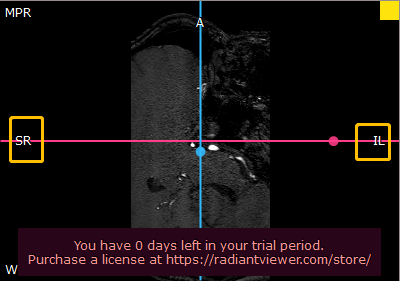# How to calculate position in MPR?

I am implementing MPR (multiplanar reformation) in my project. I have finish all the calculation except the position. For example, the RadiAnt display position as:

I understand the charact of R/L/A/P/S/I as:

``````R: right
L: left
A: Anterior
P: Posterior
S: Superior
I: Inferiro
``````

Also, dicom has a tag: ‘Patient Position’ to record the position in scanning.

My question is: how to calculate the position in MPR (`R/L/A/P/S/I`)?

Let’s say, the `Image Orientation (0020|0037)` is: `x=[cos(80°) sin(80°) 0], y=[cos(10°) sin(10°) 0], z=[0, 0, 1]`, and the `Patient Position` (0018|5100) is `HFS`.

1. Is it means that the `RL` direction is `x=[cos(80°) sin(80°) 0]`, `AP` direction is `y=[cos(10°) sin(10°) 0]`, and `SI` direction is `z=[0, 0, 1]`?

2. How to obtain the position when rotate the MPR direction? For example the following picture, how to obtain the `SR`/`IL`?In my understanding, I need to use calculate the intersection angle between the red line with `RL`/`AP`/`SI`, and find the direction with the minimum intersection angle. Is that correct?

Any suggestion is appreciated~~~